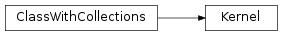# mvpa2.kernels.libsvm.Kernel¶class `mvpa2.kernels.libsvm.``Kernel`(*args, **kwargs)

Abstract class which calculates a kernel function between datasets

Each instance has an internal representation self._k which might be of a different form depending on the intended use. Some kernel types should be translatable to other representations where possible, e.g., between Numpy and Shogun-based kernels.

This class should not be used directly, but rather use a subclass which enforces a consistent internal representation, such as a NumpyKernel.

Notes

Conversion mechanisms: Each kernel type should implement methods as necessary for the following two methods to work:

`as_np()`
Return a new NumpyKernel object with internal Numpy kernel. This method can be generally inherited from the base Kernel class by creating a PrecomputedKernel from the raw numpy matrix, as implemented here.
`as_raw_np()`
Return a raw Numpy array from this kernel. This method should behave identically to numpy.array(kernel), and in fact, defining either method (via defining Kernel.__array__) will be sufficient for both method calls to work. See this source code for more details.

Other kernel types should implement similar mechanisms to convert numpy arrays to their own internal representations. See `add_conversion` for a helper method, and examples in mvpa2.kernels.sg

Assuming such `Kernel.as_*` methods exist, all kernel types should be seamlessly convertable amongst each other.

Note that kernels are not meant to be ‘functionally translateable’ in the sense that one kernel can be created, translated, then used to compute results in a new framework. Rather, the results are meant to be exchangeable, hence the standard practice of using a precomputed kernel object to store the results in the new kernel type.

For example:

```k = SomeShogunKernel()
k.compute(data1, data2)

# Incorrect and unsupported use
k2 = k.as_cuda()
k2.compute(data3, data4) # Would require 'functional translation' to the new
# backend, which is impossible

# Correct use
someOtherAlgorithm(k.as_raw_cuda()) # Simply uses kernel results in CUDA
```

Attributes

 `descr` Description of the object if any

Methods

 `add_conversion`(typename, methodfull, methodraw) Adds methods to the Kernel class for new conversions `as_ls`(kernel) `as_np`() Converts this kernel to a Numpy-based representation `as_raw_ls`(kernel) `as_raw_np`() Directly return this kernel as a numpy array `as_raw_sg`(kernel) Converts directly to a Shogun kernel `as_sg`(kernel) Converts this kernel to a Shogun-based representation `cleanup`() Wipe out internal representation `compute`(ds1[, ds2]) Generic computation of any kernel `computed`(\*args, \*\*kwargs) Compute kernel and return self `reset`()

Base Kernel class has no parameters

Attributes

 `descr` Description of the object if any

Methods

 `add_conversion`(typename, methodfull, methodraw) Adds methods to the Kernel class for new conversions `as_ls`(kernel) `as_np`() Converts this kernel to a Numpy-based representation `as_raw_ls`(kernel) `as_raw_np`() Directly return this kernel as a numpy array `as_raw_sg`(kernel) Converts directly to a Shogun kernel `as_sg`(kernel) Converts this kernel to a Shogun-based representation `cleanup`() Wipe out internal representation `compute`(ds1[, ds2]) Generic computation of any kernel `computed`(\*args, \*\*kwargs) Compute kernel and return self `reset`()
classmethod `add_conversion`(typename, methodfull, methodraw)

Adds methods to the Kernel class for new conversions

Parameters: typename : string Describes kernel type methodfull : function Method which converts to the new kernel object class methodraw : function Method which returns a raw kernel

Examples

Kernel.add_conversion(‘np’, fullmethod, rawmethod) binds kernel.as_np() to fullmethod() binds kernel.as_raw_np() to rawmethod()

Can also be used on subclasses to override the default conversions

`as_ls`(kernel)
`as_np`()

Converts this kernel to a Numpy-based representation

`as_raw_ls`(kernel)
`as_raw_np`()

Directly return this kernel as a numpy array

`as_raw_sg`(kernel)

Converts directly to a Shogun kernel

`as_sg`(kernel)

Converts this kernel to a Shogun-based representation

`cleanup`()

Wipe out internal representation

XXX unify: we have reset in other places to accomplish similar thing

`compute`(ds1, ds2=None)

Generic computation of any kernel

Assumptions:

• ds1, ds2 are either datasets or arrays,
• presumably 2D (not checked neither enforced here
• _compute takes ndarrays. If your kernel needs datasets, override compute
`computed`(*args, **kwargs)

Compute kernel and return self